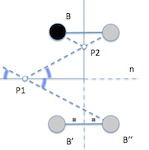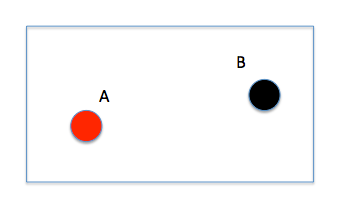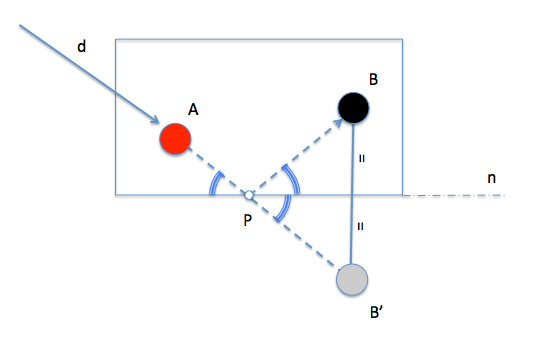# The problem with the pool table: SoluciónBy raising the problem of table, that is to hit one of the two balls that are on the table (A for example) so that it impacts the other (la B) previously given in one of the bands (Table edges), flipping the closed problem to a simple bounce case, namely, in a single band.

We can generalize the problem considering that you can give, before impact with the second ball, a given number of impacts with the bands (lateral edges) Table, but to simplify the analysis we will resolve first the simplest case: a single band.

Also assume a generalist position of the balls on the game table, so we do not have specific situations that may lead to singular solutions. The following figure shows a possible case study is outlined.The goal or solution to the problem is to determine a certain point “P” on the side, where the ball should bounce “A” before impact with the ball “B”. The address “d” therefore with which to launch the ball shall be determined by straight AP.To solve the problem we obtain the symmetric point “B” about the band “n” in which the point is “P” Searches. This symmetrical point which we will call ” B’ ” allow us to obtain the direction d as the angle formed by the straight P “n” y PB’ is the same as forming “n” and “PB” as the triangle PBB’ isosceles and straight “n” match its height above the BB side '.

Moreover we know that the angles formed by two lines and AP “n”, for example, point “P” to either side to be equal vertical angles.

In the figure these angles have been marked (equal) so that rebound comply with the rules of reflection as he proposed in the problem statement.To generalize the problem to several bands introduce a new condition on another band Bounce, the “m” for example. The solution will bring us back to symmetries to preserve the angles on the rebound of the bands. In this case we perform the symmetry of the previous case and add a new respect this band “m”. The new point ” B” ” allow us to determine the initial trajectory and get the impact point on the first rail (P1), from which solve the new item (P2) by reducing the problem to the previous model.Could you solve three-way?

GEOMETRÍA MËTRICA